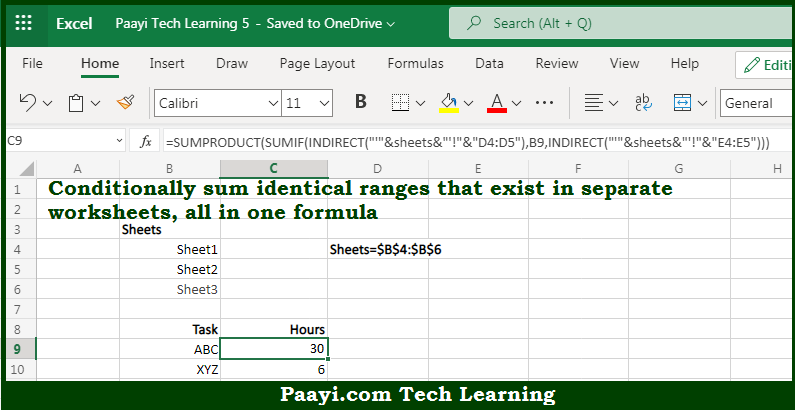# Learn How to 3D SUMIF For Multiple Worksheets in Microsoft Excel

Written by | 0 Comments | 563 Views

In this article, you will learn how to COUNT various things in Microsoft Excel using a single/combination(s) of functions. You will also know how to 3D SUMIF For Multiple Worksheets and see the generic formula.

3D SUMIF For Multiple Worksheets in Microsoft Excel

The main purpose of this formula is to conditionally sum the identical ranges that exist in separate worksheets, all in one formula. Here we will learn how to generate a 3D SUM for multiple worksheets in Microsoft Excel. That implies, with the help of a formula based on the SUMPRODUCT, SUMIF, and INDIRECT function you can able to conditionally sum the identical ranges that exist in separate worksheets, all in one formula. So, with the help of this formula, you can able to generate a 3D SUM for multiple worksheets in Microsoft Excel.

General Formula to 3D SUMIF For Multiple Worksheets

=SUMPRODUCT(SUMIF(INDIRECT("'"&sheets&"'!"&"rng"),criteria,INDIRECT("'"&sheets&"'!"&"sumrng")))

The Explanation for the 3D SUMIF For Multiple WorksheetsSo we know that with the help of the given formula above you can able to conditionally sum the identical ranges that exist in separate worksheets, all in one formula. Here we will learn how to generate a 3D SUM for multiple worksheets in Microsoft Excel. As we know that the data on all three sheets is being processed. It's the standard "3D syntax" but in case you are trying to use it with SUMIF function, you'll get a #VALUE error.  So, to solve this you can use a named range "sheets" that lists each sheet or worksheet tab that you want to include. It should be remembered that to build references to interpret correctly, you need to concatenate the sheet names to the ranges we need to work with and then use the INDIRECT to get Excel to recognize them correctly. So, with the help of this formula, you can able to conditionally sum the identical ranges that exist in separate worksheets, all in one formula.